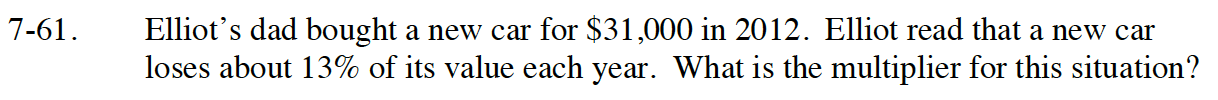Home > CC4 > Chapter 7 > Lesson 7.1.5 > Problem7-61

7-61.

Elliot’s dad bought a new car for \$31,000 in 2012. Elliot read that a new car loses about 13% of its value each year. What is the multiplier for this situation? Homework Help ✎Read Math Notes box in Lesson 5.3.2 to review the idea of multipliers.

To keep a value the same you multiply by 1.00, to decrease a value the multiplier must be less than 1.00.
If we are decreasing by 13% (0.13) what should the multiplier be?